Problem

# Solutions For Applied Combinatorics Chapter 1.2 Problem 1E

#### Step-by-Step Solution

Solution 1

To list all nonisomorphic undirected graphs with 4 vertices, do the following. First, note that an undirected graph with 4 vertices can have at most 6 edges. Now consider the cases where number of edges range from 0 to 6. For each case, draw all possible graphs, and check if any are isomorphic to any other. Keep only one graph from each set of isomorphic graphs.

Case 1: 0 edges. Clearly only one graph is possible, as there are no edges. Hence the graph is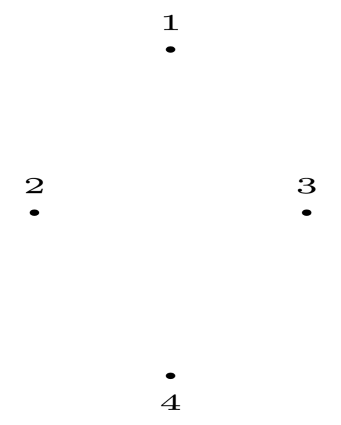Case 2: 1 edge. Again, this one edge only creates one possible graph. The graph is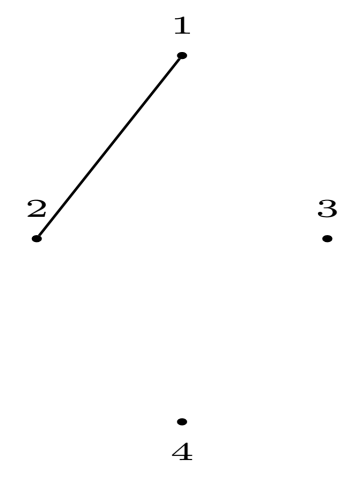Case 3: 2 edges. In this case, either the edges can share a vertex, or not. This create two cases. They are: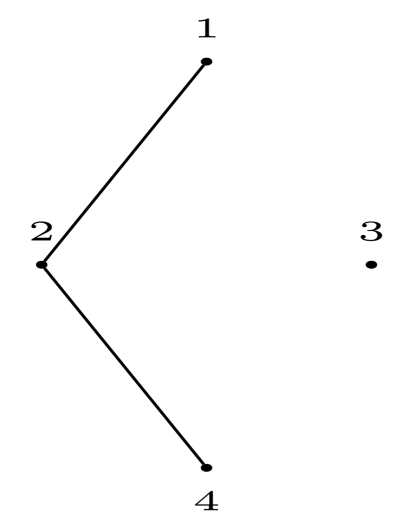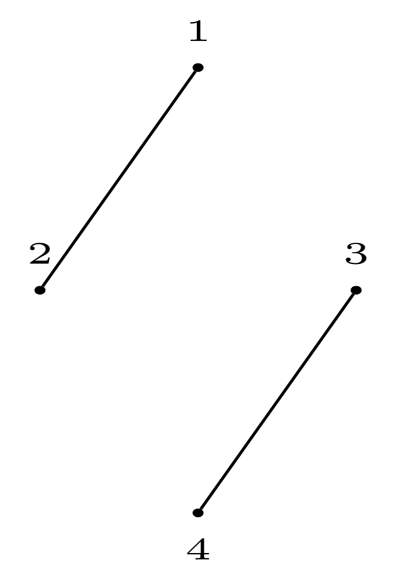Case 4: 3 edges. In this case, either they form a triangle. Or, they form a path. These are the only two possible cases. They are: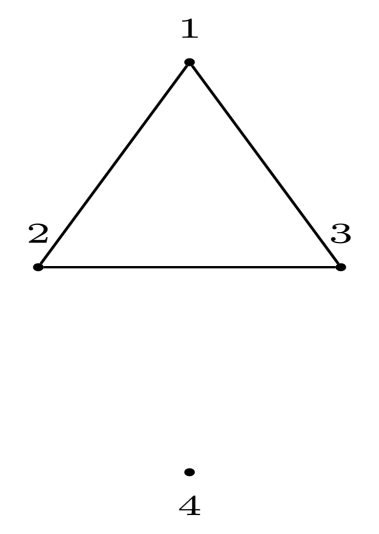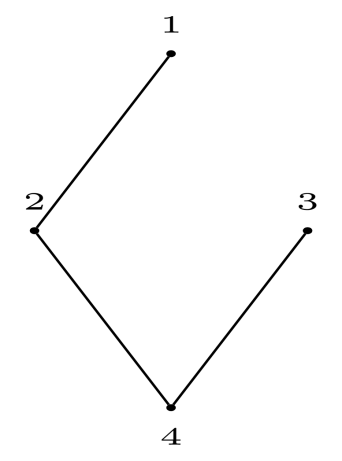Case 5: 4 edges. This case is similar to Case 3, as the 2 non-edges can be thought of as edges from Case 3. Thus, there are two possible graphs. They are: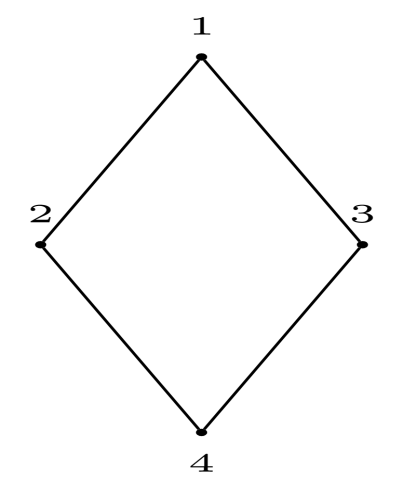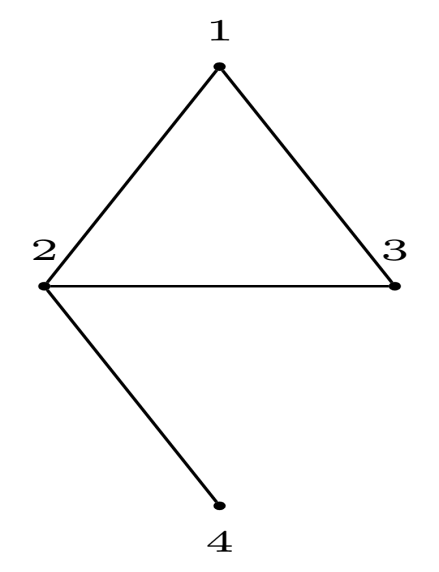Case 6: 5 edges. This is similar to Case 2, the one non-edge is similar to the one edge in Case 2. Hence there is only 1 graph. It is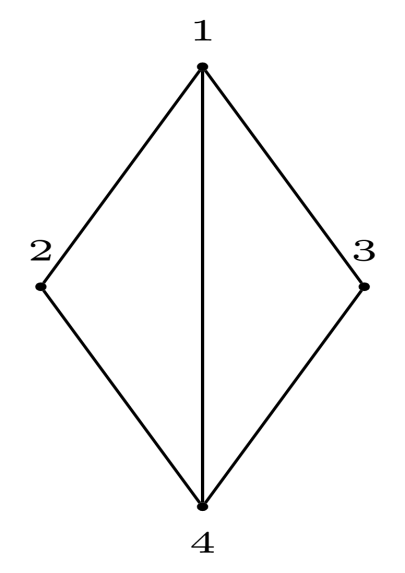Case 7: 6 edges. As all edges are present, there is only one case. It is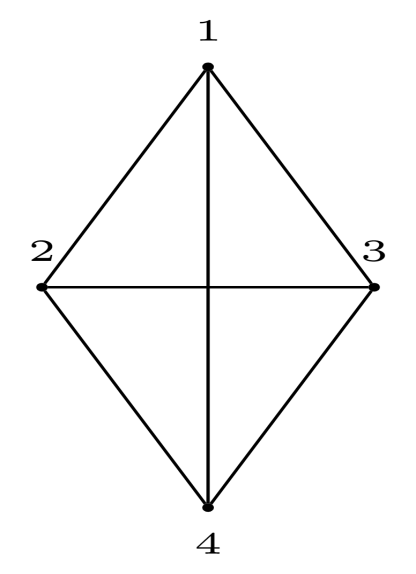These are all the possible cases.